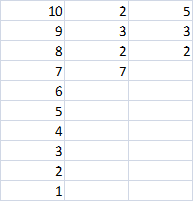﻿ Project Euler Problem 5
You are on the Home/Other Tutorials/Project Euler/Problem 5 pageShare Your

# Project Euler - Problem 5

## Problem description

2520 is the smallest number that can be divided by each of the numbers from 1 to 10 without any remainder.

What is the smallest number that is evenly divisible by all of the numbers from 1 to 20?

## Solution

We have to find the unique prime factors of each of the numbers in the list.  Unique in the sense that they do not appear in any other number already analyzed.  The easiest way to do this is to start with the largest number, list the factors, and work our way down the lower numbers.  For each, we list just the unique factors not already included because of a larger number.  So, for the list 1..10 we getMultiply the unique factors 2*5*3*3*2*2*7=2520.

Apply the same logic to the numbers from 1 to 20.